# Problem 2 Suppose two continuous random variables (X, Y) ~ f(x,y). (1) Prove E(X +Y) =...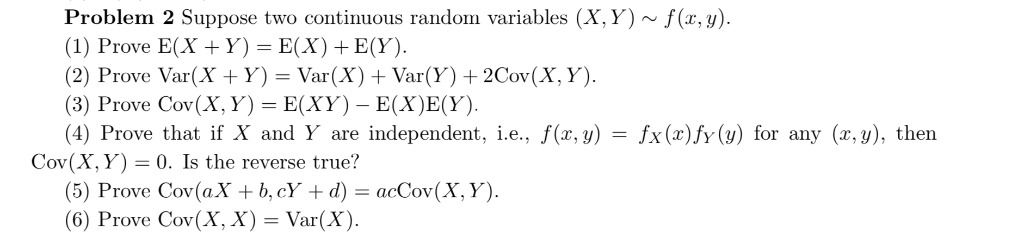Problem 2 Suppose two continuous random variables (X, Y) ~ f(x,y). (1) Prove E(X +Y) = E(X)+ E(Y). (2) Prove Var(X + Y) = Var(X) + Var(Y)2Cov(X, Y). (3) Prove Cov(X, Y) E(XY)- E(X)E(Y). (4) Prove that if X and Y are independent, i.e., f(x, y) Cov(X, Y) 0. Is the reverse true? (5) Prove Cov (aX b,cY + d) = acCov(X, Y). (6) Prove Cov(X, X) = Var(X) fx (x)fy(y) for any (x,y), then =

TOPIC:Properties of expectation,variance and covariance.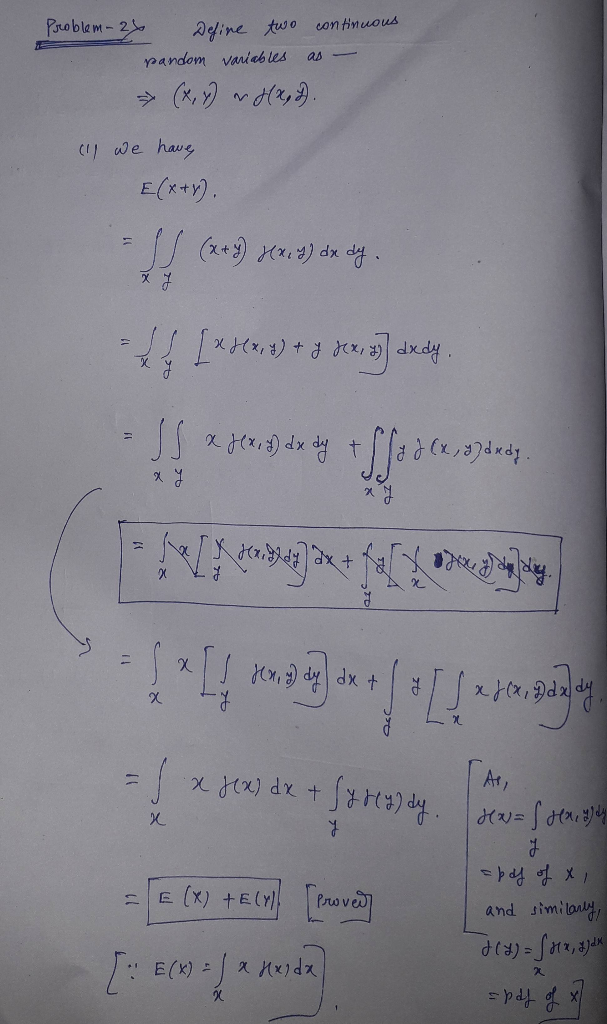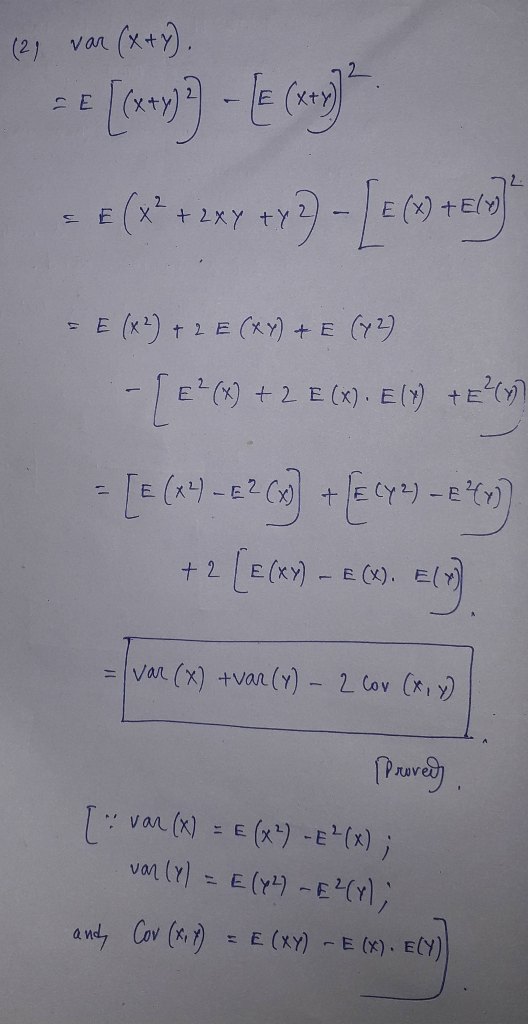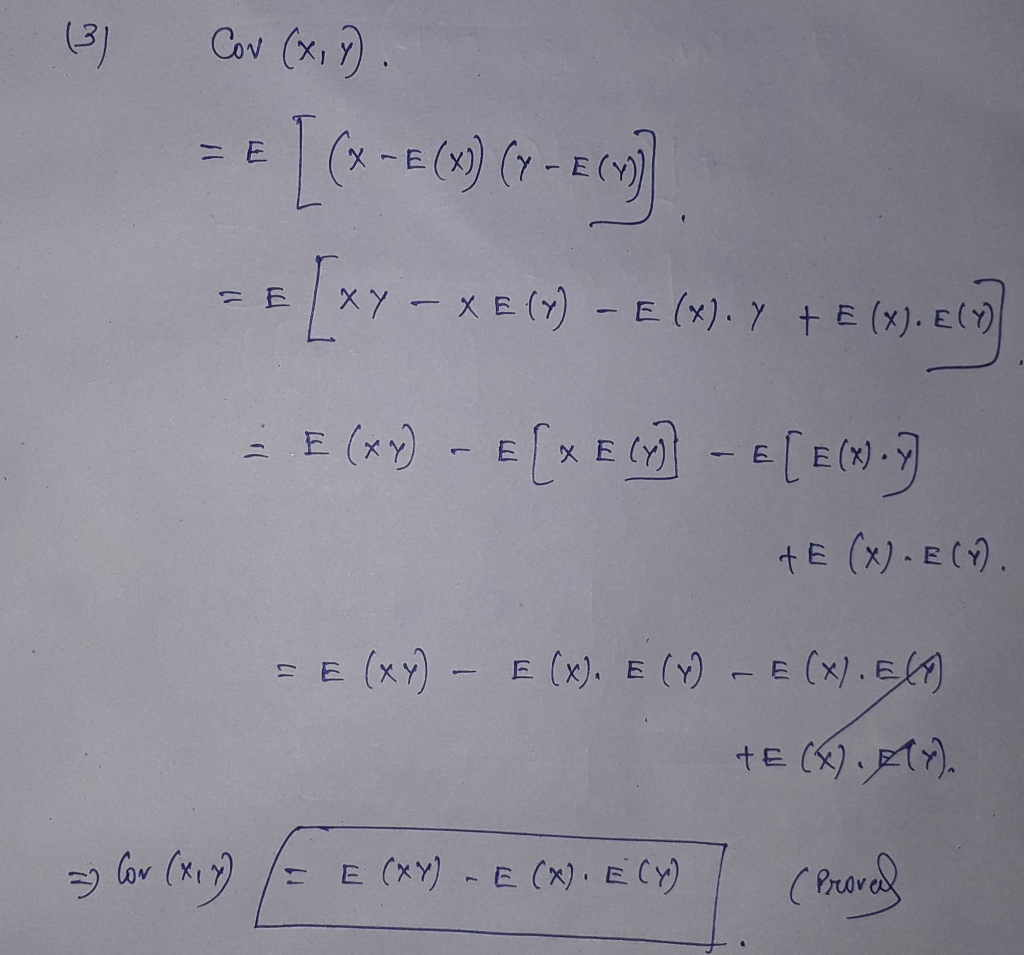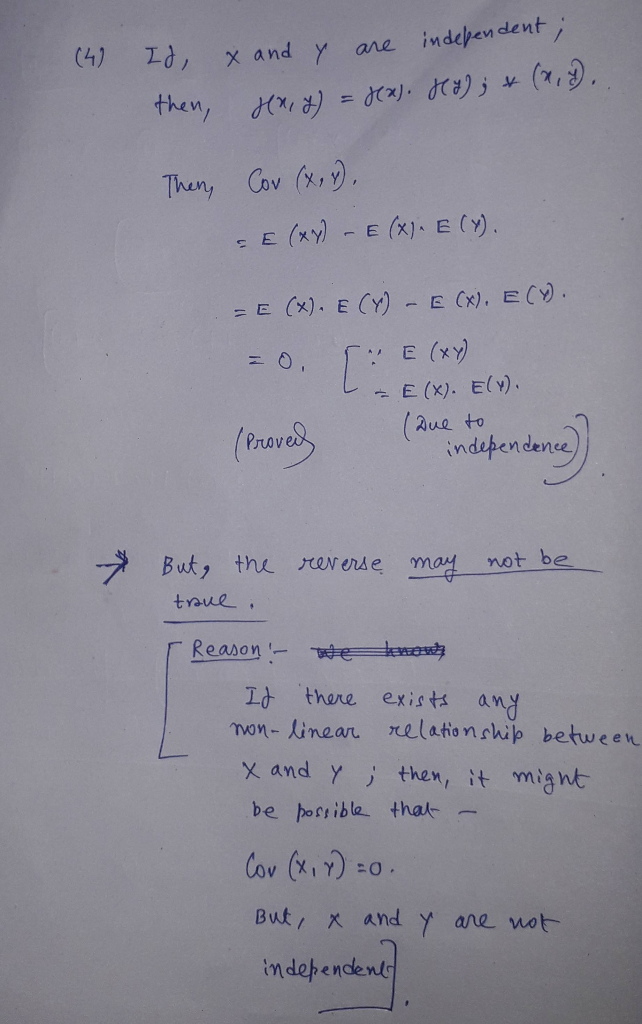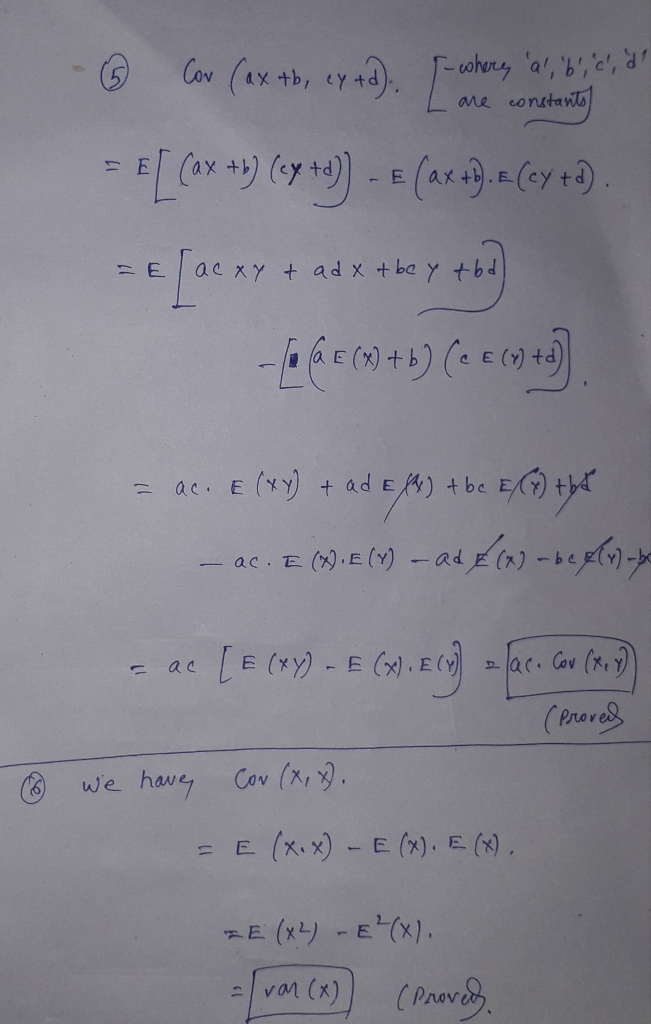##### Add Answer of: Problem 2 Suppose two continuous random variables (X, Y) ~ f(x,y). (1) Prove E(X +Y) =...
Similar Homework Help Questions
• ### For(X; Y ) ~f(x; y),where (X; Y ) is a pair of continuous random variables, and f(x,y) is the...

Prove:(1) E(X+Y)=E(x)+E(y)(2) Var(X + Y ) = Var(X) + Var(Y ) + 2Cov(X; Y ).(3)If X and Y are independent, i.e., f(x; y) = fx(x)fy(y), then Cov(X; Y ) = 0.

• ### Statistics

Please help me please. They are due on WednesdayFor two continuous random variables X and Ywith a joint density functionf(x;y), prove(1) E[X +Y ] = E[X] +E[Y ].(2) Var[X +Y ] = Var[X] +Var[Y] + 2Cov(X; Y ).(3) If X andY are independent, i.e., f(x;y) = fX(x)fY (y), thenCov(X; Y) = 0.Explain thatthereverse may not be true, i.e., if Cov(X; Y ) =0, Xand Y may not beindependent.

• ### 4. Recall that the covariance of random variables X, and Y is defined by Cov(X,Y) =...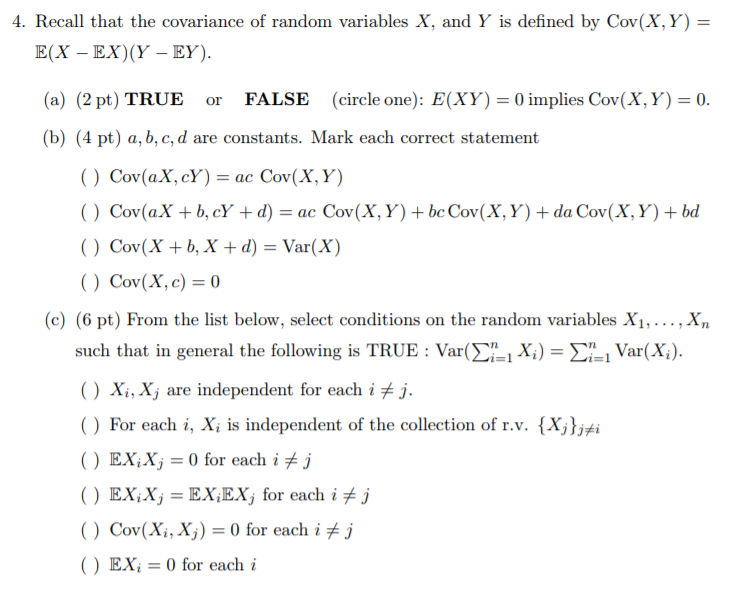4. Recall that the covariance of random variables X, and Y is defined by Cov(X,Y) = E(X - Ex)(Y - EY) (a) (2pt) TRUE or FALSE (circle one). E(XY) 0 implies Cov(X, Y) = 0. (b) (4 pt) a, b, c, d are constants. Mark each correct statement ( ) Cov(aX, cY) = ac Cov(X, Y) ( ) Cor(aX + b, cY + d) = ac Cov(X, Y) + bc Cov(X, Y) + da Cov(X, Y) + bd ( )...

• ### X and Y are random variables (a) Show that E(X)=E(B(X|Y)). (b) If P((X x, Y )...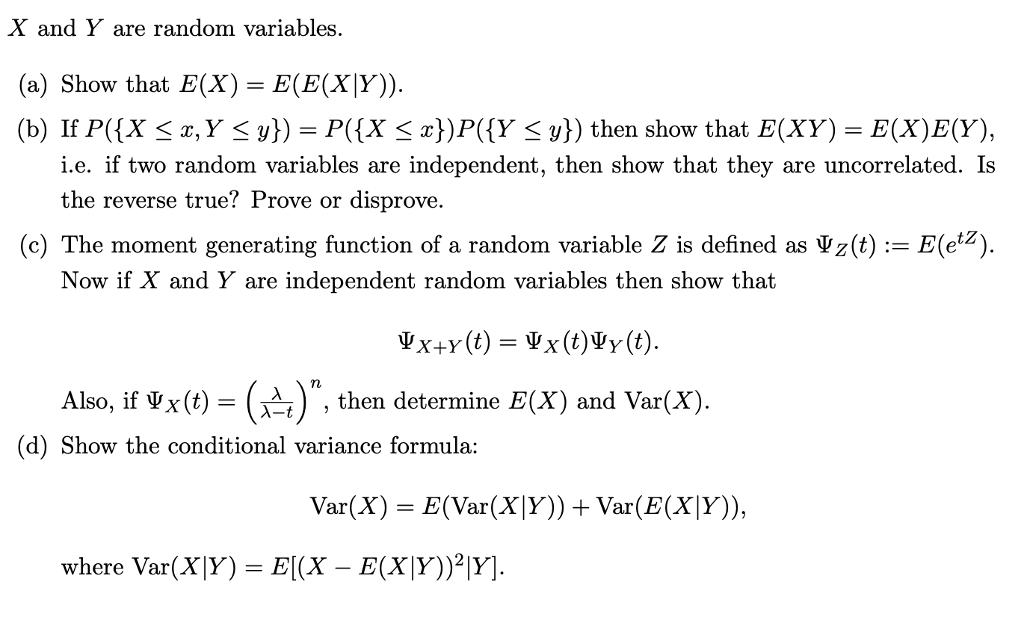X and Y are random variables (a) Show that E(X)=E(B(X|Y)). (b) If P((X x, Y ) P((X x})P({Y y)) then show that E(XY) = E(X)E(Y), i.e. if two random variables are independent, then show that they are uncorrelated. Is the reverse true? Prove or disprove (c) The moment generating function of a random variable Z is defined as ΨΖφ : Eez) Now if X and Y are independent random variables then show that Also, if ΨΧ(t)-(λ- (d) Show the conditional...

• ### 6. Suppose that X and Y are random variables such that Var(X)=Var(y)-2 and Cov(x,y)-1. the value...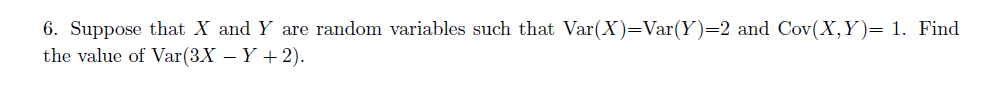6. Suppose that X and Y are random variables such that Var(X)=Var(y)-2 and Cov(x,y)-1. the value of Var(ax-y-2). Find

• ### = Var(X) and σ, 1. Let X and Y be random variables, with μx = E(X),...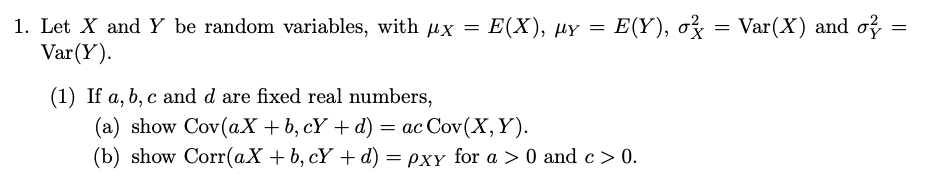= Var(X) and σ, 1. Let X and Y be random variables, with μx = E(X), μY = E(Y), Var(Y). (1) If a, b, c and d are fixed real numbers, (a) show Cov (aX + b, cY + d) = ac Cov(X, Y). (b) show Corr(aX + b, cY +d) pxy for a > 0 and c> O

• ### 1. Suppose X and Y are continuous random variables with joint pdf f(x,y) 4(z-xy) if =...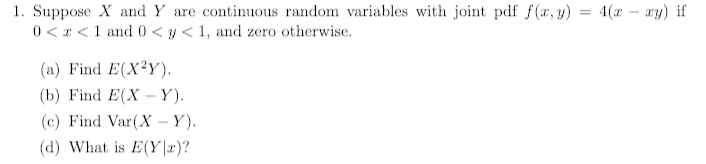1. Suppose X and Y are continuous random variables with joint pdf f(x,y) 4(z-xy) if = 0 < x < 1 and 0 < y < 1, and zero otherwise. (a) Find E(XY) b) Find E(X-Y) (c) Find Var(X - Y) (d) What is E(Y)?

• ### Let X and Y be independent identically distributed random variables with means µx and µy respectively. Prove the following. a. E [aX + bY] = aµx + bµy for any constants a and b. b. Var[X2] = E[X2] − E...

Let X and Y be independent identically distributed random variables with means µx and µy respectively. Prove the following. a. E [aX + bY] = aµx + bµy for any constants a and b. b. Var[X2] = E[X2] − E[X]2 c. Var [aX] = a2Var [X] for any constant a. d. Assume for this part only that X and Y are not independent. Then Var [X + Y] = Var[X] + Var[Y] + 2(E [XY] − E [X] E[Y]). e....

• ### Suppose X and Y are continuous random variables with joint density function 1 + xy 9...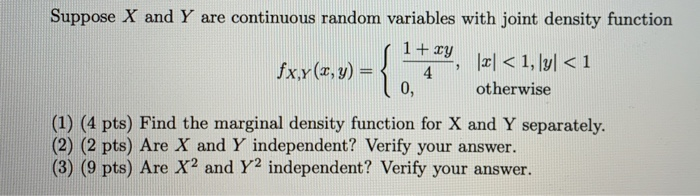Suppose X and Y are continuous random variables with joint density function 1 + xy 9 fx,y(2, y) = 4  < 1, [y] < 1 otherwise 0, (1) (4 pts) Find the marginal density function for X and Y separately. (2) (2 pts) Are X and Y independent? Verify your answer. (3) (9 pts) Are X2 and Y2 independent? Verify your answer.

• ### 2. Let X and Y be continuous random variables with joint probability density function fx,y(x,y) 0, otherwise (a) Compute the value of k that will make f(x, y) a legitimate joint probability density f...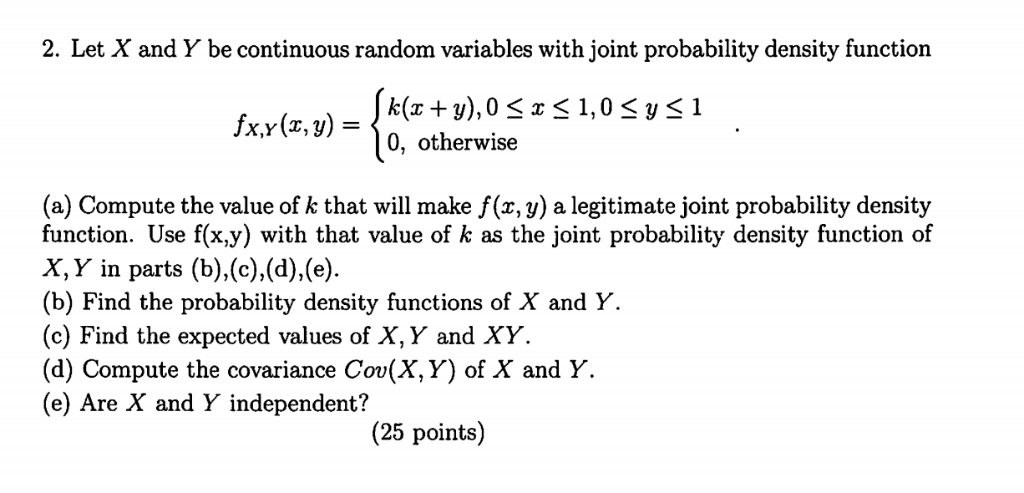2. Let X and Y be continuous random variables with joint probability density function fx,y(x,y) 0, otherwise (a) Compute the value of k that will make f(x, y) a legitimate joint probability density function. Use f(x.y) with that value of k as the joint probability density function of X, Y in parts (b),(c).(d),(e (b) Find the probability density functions of X and Y. (c) Find the expected values of X, Y and XY (d) Compute the covariance Cov(X,Y) of X...

Free Homework App